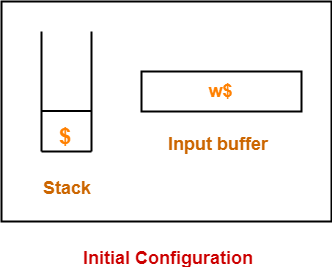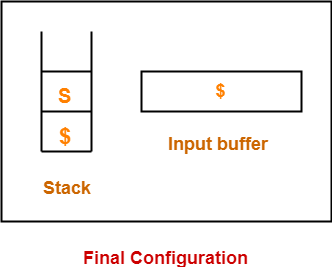## Shift-Reduce Parser-

 A shift-reduce parser is a bottom-up parser.

It takes the given input string and builds a parse tree-

• Starting from the bottom at the leaves.
• And growing the tree towards the top to the root.## Data Structures-

Two data structures are required to implement a shift-reduce parser-

• A Stack is required to hold the grammar symbols.
• An Input buffer is required to hold the string to be parsed.

## Working-

Initially, shift-reduce parser is present in the following configuration where-

• Stack contains only the \$ symbol.
• Input buffer contains the input string with \$ at its end.The parser works by-

• Moving the input symbols on the top of the stack.
• Until a handle β appears on the top of the stack.

The parser keeps on repeating this cycle until-

• An error is detected.
• Or stack is left with only the start symbol and the input buffer becomes empty.After achieving this configuration,

• The parser stops / halts.
• It reports the successful completion of parsing.

## Possible Actions-

A shift-reduce parser can possibly make the following four actions-

### 1. Shift-

In a shift action,

• The next symbol is shifted onto the top of the stack.

### 2. Reduce-

In a reduce action,

• The handle appearing on the stack top is replaced with the appropriate non-terminal symbol.

### 3. Accept-

In an accept action,

• The parser reports the successful completion of parsing.

### 4. Error-

In this state,

• The parser becomes confused and is not able to make any decision.
• It can neither perform shift action nor reduce action nor accept action.

### Rules To Remember

It is important to remember the following rules while performing the shift-reduce action-

• If the priority of incoming operator is more than the priority of in stack operator, then shift action is performed.
• If the priority of in stack operator is same or less than the priority of in stack operator, then reduce action is performed.

## Problem-01:

Consider the following grammar-

E → E – E

E → E x E

E → id

Parse the input string id – id x id using a shift-reduce parser.

## Solution-

The priority order is: id > x > –

 Stack Input Buffer Parsing Action \$ id – id x id \$ Shift \$ id – id x id \$ Reduce E → id \$ E – id x id \$ Shift \$ E – id x id \$ Shift \$ E – id x id \$ Reduce E → id \$ E – E x id \$ Shift \$ E – E x id \$ Shift \$ E – E x id \$ Reduce E → id \$ E – E x E \$ Reduce E → E x E \$ E – E \$ Reduce E → E – E \$ E \$ Accept

## Problem-02:

Consider the following grammar-

S → ( L ) | a

L → L , S | S

Parse the input string ( a , ( a , a ) ) using a shift-reduce parser.

## Solution-

 Stack Input Buffer Parsing Action \$ ( a , ( a , a ) ) \$ Shift \$ ( a , ( a , a ) ) \$ Shift \$ ( a , ( a , a ) ) \$ Reduce S → a \$ ( S , ( a , a ) ) \$ Reduce L → S \$ ( L , ( a , a ) ) \$ Shift \$ ( L , ( a , a ) ) \$ Shift \$ ( L , ( a , a ) ) \$ Shift \$ ( L , ( a , a ) ) \$ Reduce S → a \$ ( L , ( S , a ) ) \$ Reduce L → S \$ ( L , ( L , a ) ) \$ Shift \$ ( L , ( L , a ) ) \$ Shift \$ ( L , ( L , a ) ) \$ Reduce S → a \$ ( L , ( L , S ) ) ) \$ Reduce L → L , S \$ ( L , ( L ) ) \$ Shift \$ ( L , ( L ) ) \$ Reduce S → (L) \$ ( L , S ) \$ Reduce L → L , S \$ ( L ) \$ Shift \$ ( L ) \$ Reduce S → (L) \$ S \$ Accept

## Problem-03:

Consider the following grammar-

S → T L

T → int | float

L → L , id | id

Parse the input string int id , id ; using a shift-reduce parser.

## Solution-

 Stack Input Buffer Parsing Action \$ int id , id ; \$ Shift \$ int id , id ; \$ Reduce T → int \$ T id , id ; \$ Shift \$ T id , id ; \$ Reduce L → id \$ T L , id ; \$ Shift \$ T L , id ; \$ Shift \$ T L , id ; \$ Reduce L → L , id \$ T L ; \$ Shift \$ T L ; \$ Reduce S → T L \$ S \$ Accept

## Problem-04:

Considering the string “10201”, design a shift-reduce parser for the following grammar-

S → 0S0 | 1S1 | 2

## Solution-

 Stack Input Buffer Parsing Action \$ 1 0 2 0 1 \$ Shift \$ 1 0 2 0 1 \$ Shift \$ 1 0 2 0 1 \$ Shift \$ 1 0 2 0 1 \$ Reduce S → 2 \$ 1 0 S 0 1 \$ Shift \$ 1 0 S 0 1 \$ Reduce S → 0 S 0 \$ 1 S 1 \$ Shift \$ 1 S 1 \$ Reduce S → 1 S 1 \$ S \$ Accept

To gain better understanding about Shift-Reduce Parsing,

Watch this Video LectureNext Article- Operator Precedence Parsing

Get more notes and other study material of Compiler Design.

Watch video lectures by visiting our YouTube channel LearnVidFun.